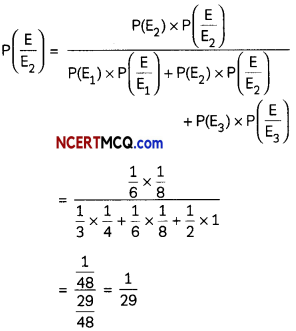Students can access the CBSE Sample Papers for Class 12 Maths with Solutions and marking scheme Term 2 Set 3 will help students in understanding the difficulty level of the exam.

## CBSE Sample Papers for Class 12 Maths Term 2 Set 3 with Solutions

Time Allowed: 2 Hours
Maximum Marks: 40

General Instructions:

• This question paper contains three sections-A. B and C. Each part is compulsory.
• Section-A has 6 short answer type (SA1) questions of 2 marks each.
• Section-B has 4 short answer type (SA2) questions of 3 marks each.
• Section-C has 4 long answer type questions (LA) of 4 marks each.
• There is an Internal choice in some of the questions.
• Q14 is a case-based problem having 2 sub parts of 2 marks each.

Section – A
(Section – A has 6 short answer type (SA-1) questions of 2 marks each.)

Question 1.
Evaluate ∫$$\frac{2^{x+1}-5^{x-1}}{10^{x}}$$ dx. (2)
Let I = ∫$$\frac{2^{x+1}-5^{x-1}}{10^{x}}$$ dx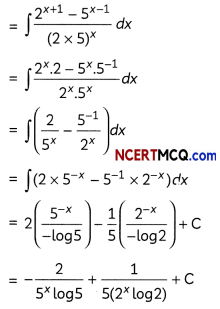Question 2.
A line makes angle α, β, γ with the coordinate axes. If α + β = 90°, then find the value of γ. (2)
If a tine makes angles α, β, γ with coordinate axes, then we have,
cos2 α + cos2β + cos2γ = 1
Since, α + β = 90°
⇒ α = 90° – β
⇒ cos α = cos (90° – β)
⇒ cos α = sin β
⇒ cos2α = sin2β
= 1 – cos2β
⇒ cos2α + cos2β = 1
From (i) and (ii), we get
⇒ 1 + cos2γ = 1
⇒ cos2γ = 0
Hence, γ = $$\frac{\pi}{2}$$ = 90°Question 3.
Find the probability of the occurrence of a number greater than 2 in a throw of a die, if it is known that only even numbers can occur.
OR
An unbaised die is thrown. If a random variable X is defined asWrite the probability distribution of X. (2)
Let A be the event of “occurrence of an even number”, and B be the event of “occurrence of a number greater than 2.
∴ P(A) = $$\frac{3}{6}=\frac{1}{2}$$
And P(B n A) = $$\frac{2}{6}=\frac{1}{3}$$
[∵ 4 and 6 are even numbers from 1 to 6 that are greater than 2]
Now,
Required probability = P(B/A)
= $$\frac{P(B \cap A)}{P(A)}$$
= $$\frac{1 / 3}{1 / 2}=\frac{2}{3}$$
OR
Here, X takes the values 0 and 1.
Also, P(X = 0) = P(an odd number = $$\frac{3}{6}=\frac{1}{2}$$
And, P(X = 1) = P(an even number) = $$\frac{3}{6}=\frac{1}{2}$$
Thus, the probability distribution of X is: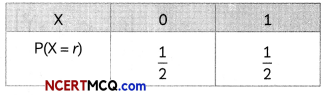Question 4.
If $$\vec{a}$$ = 5î – ĵ – 3k̂ and $$\vec{b}$$ = î + 3ĵ – 5k̂, then show that $$(\vec{a}+\vec{b})$$ and $$(\vec{a}-\vec{b})$$ are orthogonal. (2)
We have,
$$\vec{a}+\vec{b}$$ = (5î – ĵ – 3k̂) + (î + 3ĵ – 5k̂)
= 6î + 2ĵ – 8k̂
and $$\vec{a}-\vec{b}$$ = (5î – ĵ – 3k̂) – (î + 3ĵ – 5k̂)
= 4î – 4ĵ + 2k̂

Now, $$(\vec{a}+\vec{b})$$. $$(\vec{a}-\vec{b})$$ = (6î + 2ĵ – 8k̂) . (4î – 4ĵ + 2k̂)
= 6 × 4 + 2 × (- 4) + (- 8) × 2
= 24 – 8 – 16
= 0

Hence, $$(\vec{a}+\vec{b})$$ and $$(\vec{a}-\vec{b})$$ are orthogonal i.e., perpendicular to each other.

Question 5.
Check whether the differential equation (x – y) $$\frac{d y}{d x}$$ = x + 2y is homogenous or not. (2)
We have,Since, the power of λ is zero.
∴ The given differential equation is homogeneous.

Question 6.
Bag I contains 2 white and 4 red balls. Bag II contains 3 white and 3 red balls. One of the bags is selected at random and a ball is drawn from it. If the ball drawn is white, what is the probability that it is drawn from Bag I? (2)
Let E1 and E2 be the events of selecting Bag I and Bag II respectively.
Also, let W be the event of drawing a white ball. Then,
P(E1)= $$\frac{1}{2}$$ and P(E2) = $$\frac{1}{2}$$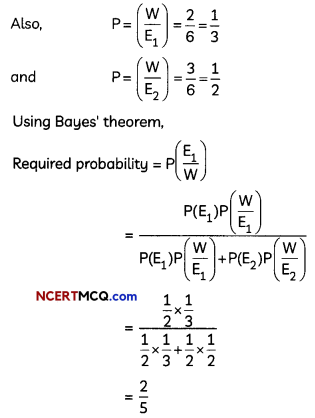Section – B
(Section – B has 4 short answer type (SA-2) questions of 3 marks each.)

Question 7.
Evaluate ∫tan-1(secx + tanx)dx.
OR
Evaluate ∫01 $$\frac{1}{\sqrt{a x-x^{2}}}$$ dx. (3)
Let ∫tan-1(secx + tanx)dx.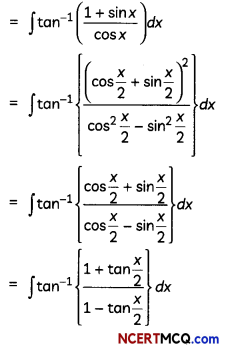[Dividing numerator and denominator by $$\frac{x}{2}$$]Question 8.
Find the general solution of y2 dx + (x2 – xy + y2) dy = 0. (3)
Given, diffrentiaL equation is
y2dx + (x2 – xy + y2)dy =0
⇒ y2dx = -(x2 – xy + y2)dy
⇒ y2 $$\frac{d x}{d y}$$ = -(x2 – xy + y2)
⇒ $$\frac{d x}{d y}=-\left(\frac{x^{2}}{y^{2}}-\frac{x}{y}+1\right)$$ ……(i)
This is a homogeneous differential equation.

Let x = vy …….(ii)
$$\frac{d x}{d y}$$ = v + y$$\frac{d v}{d y}$$
Substituting these values in equation (i), we get
v + y$$\frac{d v}{d y}$$ = -(v2 – v + 1)
⇒ y$$\frac{d v}{d y}$$ = -v2 – 1
⇒ $$\frac{d v}{d y}$$

On integrating both sides, we get
tan-1(y) = -log y + c
⇒ tan-1 + log y = c [From (ii)]

Caution:
Here, we are differentiating x w.r.t y so we will use x = vy for substitution.

Question 9.
Find the distance between the parallel planes 6x + 2y – 3z = 12 and 12x + 4y – 6z = 17. (3)
Given: Equation of ptanes ore:
6x + 2y – 3z = 12 ………(i)
and 12x + 4y – 6z = 17
or 6x + 2y – 3z = $$\frac{17}{2}$$
∵ Planes (1) and (ii) are paralleL

∴ Distance between them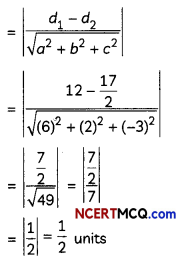Related Theory:
Distance between two parallel planes ax + by + cz = d1 and ax + by + cz = d2 is given as $$\left|\frac{d_{1}-d_{2}}{\sqrt{a^{2}+b^{2}+c^{2}}}\right|$$

Question 10.
Let the vectors $$\vec{a}, \vec{b}, \vec{c}$$ be given as a1x î + a2ĵ + a3 k̂, b1î + b2ĵ + b3k̂ and c1î + c2ĵ + c3k̂ respectively. Then show that $$\vec{a} \times(\vec{b}+\vec{c})=(\vec{a}+\vec{b})+(\vec{a} \times \vec{c})$$
OR
Find the area of the triangle whose vertices are A (1,1,2), B (2, 3, 5) and C (1, 5, 5). (3)
We have,
$$\vec{b}+\vec{c}$$ = (b1î + b2ĵ + b3k̂) + (c1î + c2ĵ + c3k̂)
= (b1 + c1)î + (bsub>2 + csub>2)ĵ + (b3 + c3) k̂= {a2 (b3 + c3) – a3 (b2 + c2)}î – {a1 (b3 + c3) – a3(b1 + c1}ĵ + {a1(b2 + c2) – a2(b1 + c1)k̂So $$(\vec{a} \times \vec{b})+(\vec{a} \times \vec{c})$$ = (a2b3 – a3b2)î – (a1b3 – a3b1)ĵ + (a1b2 – a2b1) k̂ + (a1c3 – a3c1) î -(a1c3 – a3c1)ĵ + (a1c2 – a2c1) k̂
= {a2(b3 + c1) – a3(b2 + c2)} î – {a1(b3 + c3) – a3(b1 + c1}ĵ + {i (b2 + c2) – 2 (b1 + c1)} k̂ …(iv)

From (i) and (iv), we get
$$\vec{a} \times(\vec{b}+\vec{c})=(\vec{a}+\vec{b})+(\vec{a} \times \vec{c})$$
Hence proved.

OR

We have, $$\overrightarrow{\mathrm{AB}}$$ = (2 – 1)î + (3 – 1)ĵ + (5 – 2) k̂
= î + 2ĵ + 3k̂

and $$\overrightarrow{\mathrm{AC}}$$ = (1 – 1)î + (5 – 1)ĵ +(5 – 2)k̂
= 4ĵ +3k̂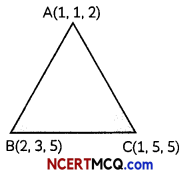We know,
Area of ∆ABC = $$\frac{1}{2}|\overrightarrow{A B} \times \overrightarrow{A C}|$$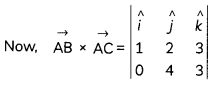= (6 – 12)î – (3 – 0)ĵ + (4 – 0)k̂ – 6î – 3ĵ + 4k̂
∴$$|\overrightarrow{A B} \times \overrightarrow{A C}|=\sqrt{(-6)^{2}+(-3)^{2}+4^{2}}$$
= $$\sqrt{36+9+16}$$
= $$\sqrt{61}$$
∴ Area of ∆ABC = $$\frac{1}{2}|\overrightarrow{A B} \times \overrightarrow{A C}|=\frac{\sqrt{61}}{2}$$sq. units

Section – C
(Section – C has 4 Long answer type questions (LA) of 4 marks each.)

Question 11.
Evaluate ∫$$\frac{1}{3+2 \sin x+\cos x}$$ dx
OR
Evaluate ∫0π/2$$\frac{\cos x}{1+\cos x+\sin x}$$ dx. (4)
Let I = ∫$$\frac{1}{3+2 \sin x+\cos x}$$ dx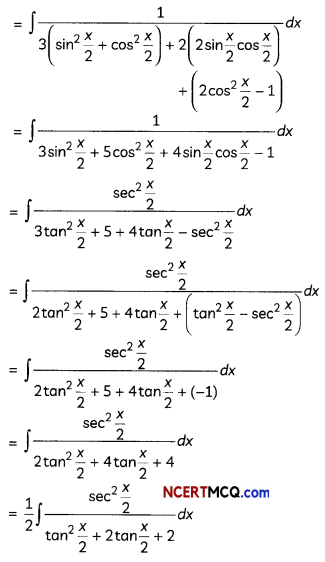Put tan $$\frac{x}{2}$$ = t
⇒ $$\frac{1}{2}$$sec2$$\frac{x}{2}$$ dx = dt
⇒ sec2$$\frac{x}{2}$$ dx = 2dt
∴ I = ∫$$\frac{d t}{t^{2}+2 t+2}$$
= ∫$$\frac{d t}{(t+1)^{2}+1}$$
= tan-1(t + 1) + C
[∵∫$$\frac{1}{x^{2}+a^{2}}$$dx = $$\frac{1}{a}$$tan-1$$\frac{x}{a}$$
= tan-1(tan$$\frac{x}{2}$$ + 1) + C
OR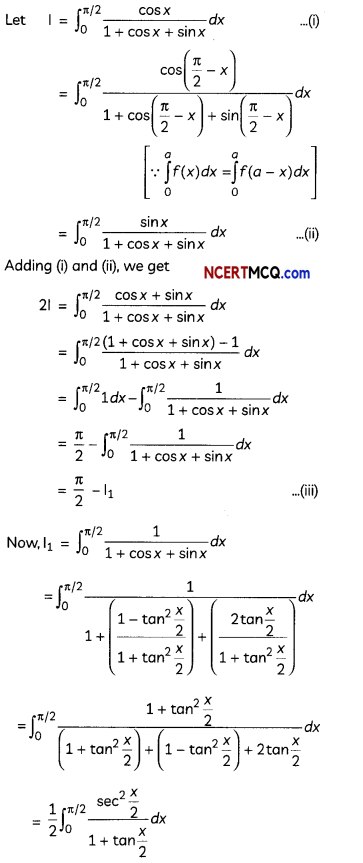Put 1 + tan$$\frac{x}{2}$$ = t
⇒ $$\frac{1}{2}$$sec2 $$\frac{x}{2}$$dx = dt
or sec2 $$\frac{x}{2}$$dx = 2dt
Also, when x = 0, t = 1
when x = $$\frac{π}{2}$$ t =2
∴ I1 = ∫12$$\frac{d t}{t}$$
= [log t]12
= log 2

Substituting the value of I1 in (iii), we get
2I = $$\frac{π}{2}$$ – Log 2
⇒ I = $$\frac{π}{2}$$ – $$\frac{1}{2}$$Log 2Question 12.
Find the area bounded by the curve y = √x, x = 2y + 3 in the first quadrant and x-axis. (4)
Given: y= √x and x = 2y + 3 in the first quadrant
Putting x = 2y + 3 in y = √x we get
y = $$\sqrt{2 y+3}$$
⇒ y2 = 2y+3
⇒ y2 – 2y – 3 = 0
⇒ y2 – 3y + y – 3 = 0
⇒ y(y – 3) + 1(y – 3) = 0
⇒ (y + 1)(y – 3) = 0
⇒ y = -1, 3
∴ x = 1.9
∴ Points of intersection of the given curve and the line are (1, -1) and (9, 3).∴ Required area of shaded region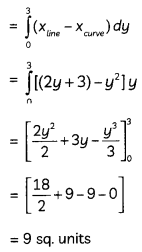Question 13.
If î + ĵ + k̂, 2î +5ĵ, 3î + 2 ĵ – 3k̂ and î – 6ĵ – k̂ are the position vectors of points A, B, C and D respectively, then find the angle between the vectors $$\overrightarrow{\mathrm{AB}}$$ and $$\overrightarrow{\mathrm{CD}}$$. (4)
We have, $$\overrightarrow{\mathrm{AB}}$$ = (2 – 1)î + (5 – 1)ĵ + (0 – 1)k̂
= î + 4ĵ – k̂
And
$$\overrightarrow{\mathrm{CD}}$$ = (1 – 3)î +(-6 – 2) + (-1 + 3)k̂
= -2î – 8ĵ + 2k̂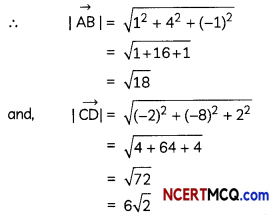Let θ be the angle between the vectors $$\overrightarrow{\mathrm{AB}}$$ and $$\overrightarrow{\mathrm{CD}}$$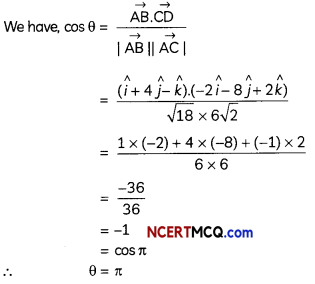Hence the angle between the vectors $$\overrightarrow{\mathrm{AB}}$$ and $$\overrightarrow{\mathrm{CD}}$$ is π.Case-Based/Data-Based

Question 14.
In an examination, an examinee either guesses or copies or knows the answer of MCQs with four choices. The probability that he makes a guess is $$\frac{1}{3}$$, and the probability that he copies answer is $$\frac{1}{6}$$. The probability that his answer is correct given that he copied it, is $$\frac{1}{8}$$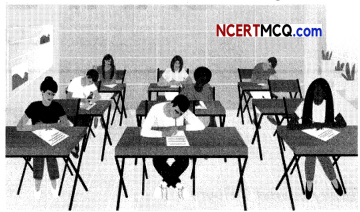Based on the above information, answer the following two questions:
(A) What is the probability that he answers the question correctly? (2)
Let E1, E2, E3, and E be the events defined as follows:
E: Examinee answers the question correctly.
∴ P(E1) = $$\frac{1}{3}$$
P(E2) = $$\frac{1}{6}$$
P(E3) = 1 – $$\left(\frac{1}{3}+\frac{1}{6}\right)=\frac{1}{2}$$

Also, = (Since, a question has four choices, out of which only one is correct.)
P$$\left(\frac{E}{E_{2}}\right)=\frac{1}{8}$$
P$$\left(\frac{E}{E_{3}}\right)$$ = 1

(When the student knows the answer, the probability of his answer being correct is 100%.)

(A) P(E) = P(E1) × P$$\left(\frac{E}{E_{1}}\right)$$ + P$$\left(\frac{E}{E_{2}}\right)$$ + P(E3) × P$$\left(\frac{E}{E_{3}}\right)$$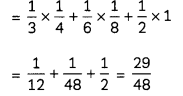(B) Given that he answered the question correctly, what is the probability that he copied the answer? (2)